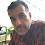## Monday, December 12, 2016

### Flattened logistic regression vs. empirical logit

I first learned about quasi-logistic regression and the "emprical logit" from Dale Barr's (2008) paper, which just happened to be right next to the growth curve analysis paper that Jim Magnuson, J. Dixon, and I wrote. I came to understand and like this approach in 2010 when Dale and I co-taught a workshop on analyzing eye-tracking data at Northwestern. I give that background by way of establishing that I'm positively disposed to the empirical logit method. So I was interested to read a new paper by Seamus Donnelly and Jay Verkuilen (2017) in which they point out some weaknesses of this approach and offer an alternative solution.

In short, the problems raised by Donnelly and Verkuilen (D&V) are that the empirical logit transformation tends to bias proportion estimates toward 0.5 (i.e., logit=0) and that the likelihood function is different. These don't seem like particularly controversial claims. To me, biasing toward 0.5 and using a Gaussian likelihood function are rather the point of using the empirical logit -- the biasing helps counteract the effects of floor and ceiling values that can arise in psychology experiments (i.e., 100% or 0% accuracy can be observed with a limited number of trials even when a participant is not actually that perfect) and the Gaussian likelihood function helps with model convergence. However, D&V offer an alternative approach, flattened logistic regression, and show using simulations that it works better.

I tried it out on some of my data and the results were not very clear (the example data and helper functions are from psy811, a little package I wrote for my multilevel regression course, the main model-fitting functions are from lme4).

library(psy811)
#compute values for logistic regression
WordLearnEx\$NumCorr <- round(WordLearnEx\$Accuracy*6)
WordLearnEx\$NumErr <- 6 - WordLearnEx\$NumCorr
#compute empirical logit values
WordLearnEx\$elog <- with(WordLearnEx, log((NumCorr+0.5)/(NumErr+0.5)))
WordLearnEx\$wts <- with(WordLearnEx, 1/(NumCorr+0.5) + 1/(NumErr+0.5))
#set up orthogonal polynomial
WordLearn <- code_poly(WordLearnEx, predictor = "Block", poly.order=2, draw.poly = FALSE)
#fit elog model
m.elogit <- lmer(elog ~ (poly1+poly2)*TP + (poly1+poly2 | Subject), data=WordLearn, weights=1/wts, REML=FALSE)
get_pvalues(m.elogit)
#fit logistic regression
m.log <- glmer(cbind(NumCorr, NumErr) ~ (poly1+poly2)*TP + (poly1+poly2 | Subject), data=WordLearn, family="binomial")
coef(summary(m.log))
#fit flattened logistic regression
#Flat = 0.1

m.flog1 <- glmer(cbind(NumCorr+0.1, NumErr+0.1) ~ (poly1+poly2)*TP + (poly1+poly2 | Subject), data=WordLearn, family="binomial")
coef(summary(m.flog1))

The empirical logit and logistic regression versions converged fine, but the flattened logistic regression gave a convergence warning ("Model failed to converge with max|grad| = 0.191469 (tol = 0.001, component 1)"), in addition to the expected warning about non-integer values in a binomial glm. On the other hand, the pattern of results was very similar across these three models; even the parameter estimates for the two logistic models were very similar. I also tried a few other flattening constants (as recommended by D&V), and the results were basically the same -- same convergence warning, essentially the same parameter estimates.

So I'm not quite sure what to think; I'll keep trying it on other data sets as opportunities come up. In general, my preference is to stick with standard logistic regression and steer away from alternatives like empirical logit analysis. When that fails, I'm not sure which is the next best option -- the flattened logistic approach looks promising from the D&V simulations, but I'd like to try it some more on my own data.Donnelly, S., & Verkuilen, J. (2017). Empirical logit analysis is not logistic regression Journal of Memory and Language, 94, 28-42 DOI: 10.1016/j.jml.2016.10.005
Barr, D. (2008). Analyzing ‘visual world’ eyetracking data using multilevel logistic regression Journal of Memory and Language, 59 (4), 457-474 DOI: 10.1016/j.jml.2007.09.002

1.2.3.